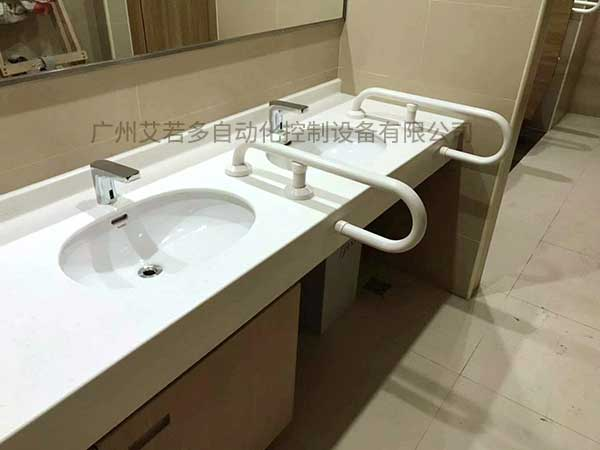首页 »

»

# 进口卫生间扶手

• 产品详情I型NS-T400L=400mmφ34mm

NS-T600L=600mm

NS-T800L=800mm

L型NS-T7060CL

(向左)L=700×600mm

NS-T7060CR

(向右)

NS-T7070L=700×700mm

(设置于墙壁)―

NS-TRF

(设置于地板)

(纵向可动式)L=700mm(规格)

L=850mm(准规格)

NS-CM4

(纵向可动式)L=700mm(规格)

L=600mm(准规格)

NS-CM1n

(横向可动式)L=700mm(规格)

L=850mm(准规格)

NS-LC1L=550mm

NS-LC2L=700mm

H=700mm

H=600mm

W=600mm

H=440mm

NS-LC2L=700mm

H=760mm

NS-L1L=700mm

W=700mm

H=760mm

NS-S1L=580mm